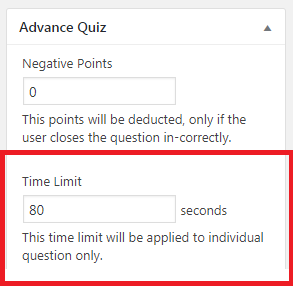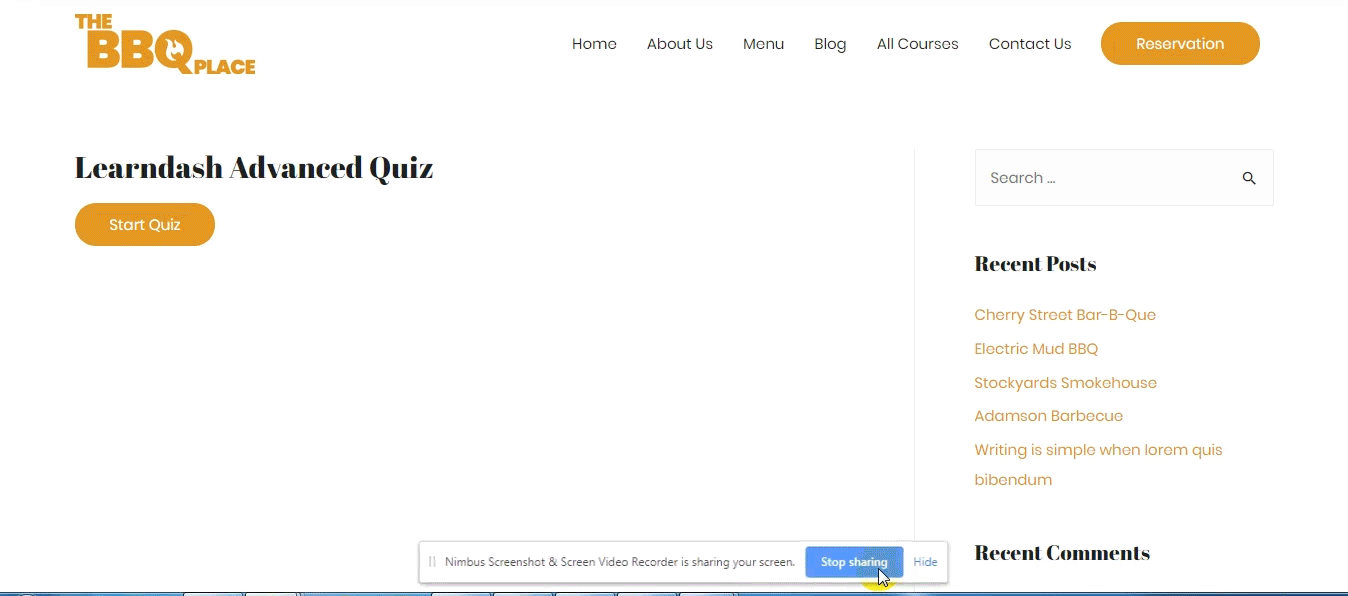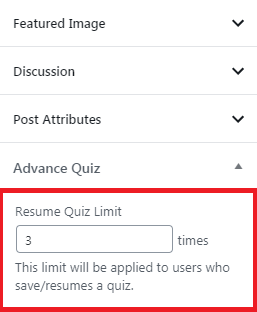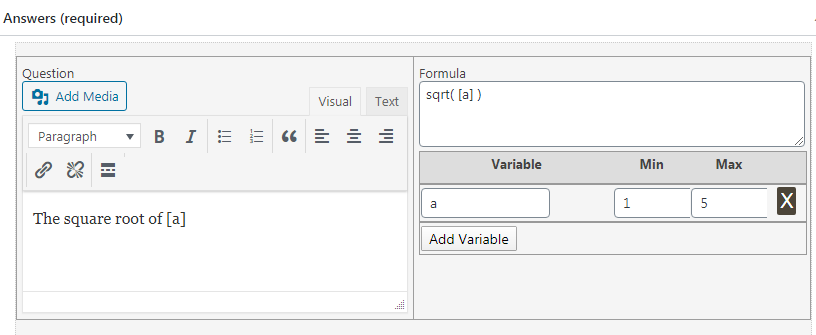# To enable time limits for your quiz questions, you must check the option of Different time limits for each question from the Advanced Quiz General Settings menu. You can find this section by selecting LearnDash from the WordPress dashboard and then selecting Advanced Quiz. Finally, click on the Update Settings button to save your changes. You can set the time limit for each question on the question edit page from the meta box on the right.## Save/Resume Quiz:

Navigate to LearnDash from the WordPress dashboard and select Advanced settings. Next, check the box next to  Allow Save/Resume Quiz in the General Settings tab. Finally, select Update Settings to save changes and enable the feature.When this feature is enabled, your answers will be saved after saving a quiz, and you will be able to resume the evaluation with the answers you saved previously on your next visit to that quiz.

Upon resuming the quiz, you will be taken to the same question from where you left off. Your quiz state will be saved and if you have answered any previous question that answer will also be saved there too, for the ease of the students/users to get back to it whenever they feel like resuming their attempt.

### Resume limit:

If you want to limit the number of times your students can resume work on a quiz, you can also set a ”resume quiz limit”  from the meta box on the right side on the quiz edit page.# Square root, cos, sin, tan, abs, exp, log, round, ceil, floor, fmod functions, and all the mentioned functions below are supported in the calculated formula question type.### Functions Format:

Use the following format to use functions in the calculated formula question type.

function(   [a]  )

Here “a” is the variable that contains a value.

• cos( [a] )
• sin( [a] )
• tan( [a] )
• abs( [a] )
• exp( [a] )
• log( [a] )
• sqrt( [a] )
• int( [a] )
• round( [a] )
• ceil( [a] )
• floor( [a] )
• fmod( [a] )
• acos( [a] )
• acosh( [a] )
• asin( [a] )
• asinh( [a] )
• atan2( [a] )
• atan( [a] )
• atanh( [a] )
• bindec( [a] )
• cosh( [a] )
• decbin( [a] )
• dechex( [a] )
• decoct( [a] )
• hexdec( [a] )
• log10( [a] )
• max( [a] )
• min( [a] )
• octdec( [a] )
• pi( [a] )
• rand( [a] )
• sinh( [a] )
• rand( [a] )
• srand( [a] )
• tanh( [a] )

# Developer’s Doc:

## Action and Filter Hooks:

Purpose: Modifies the “Save” button text.

Parameters:

• \$button_text (string)
• \$quiz_post_id (integer)

Purpose: Modifies the “Resume” button text.

Parameters:

• \$button_text (string)
• \$quiz_post_id (integer)